## General Mechanical

•Venugopalb
Subscriber

Hello all!

I am doing nonlinear buckling analysis in an ellipsoidal dome structure. for that i did linear buckling analysis and calculated the linear buckling load through the load factor. Now I started to do nonlinear analysis. Here i used Arclength method to get solution. After getting some results from this Arclength method, I don't know how to calculate the nonlinear  buckling pressure for the dome structure(Pressure vs Displacement curve). The dome structure is loaded with pressure on its outer surface.

•peteroznewman
Subscriber

You have a time history for the solution which has a value of Pressure and Displacement at each time step.  You can copy a table of each of those variables out of Mechanical and paste them in Excel to plot the Pressure vs Displacement curve.  You can do it in Mechanical also, I just find it easier in Excel.

•jj77
Subscriber

In ARCLEN it will scale pressure as needed so one just applies the full load in one step. Peter do you say that there is a way to still get the actual applied pressure in this case or were you referring to when you have applied a time table with the pressure yourself, where in that case of course the pressure is known at different times?

The time steps taken by ARCLEN are somehow related to the applied pressure, but I am not really 100 % on this. So do not trust that fully

•peteroznewman
Subscriber

I haven't used ARCLEN so perhaps I was wrong about the availability of the applied pressure increments during the substeps that ramp up to the full load.

•peteroznewman
Subscriber

Here is a 2D Dome with a Pressure load at the center of 9 Pa applied in a single 1 second Time Step.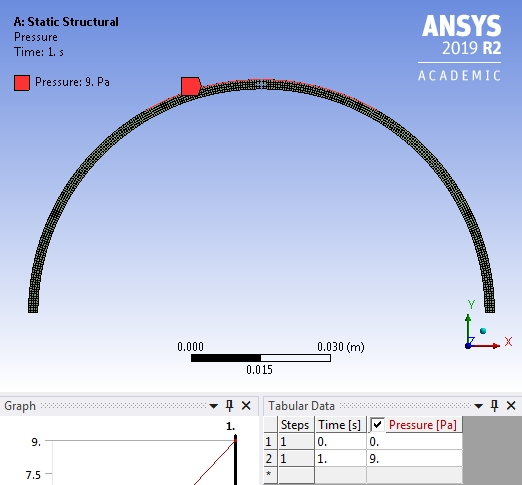Here is the Deformation Result in mm and the tabular data of Time and Displacement.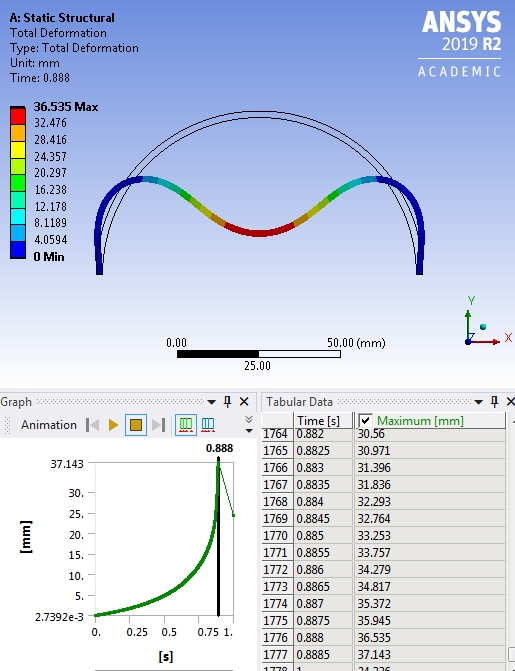I copy the tabular data to Excel, and insert a column called Pressure, then use a formula, Pressure = 9*Time.

Here is the Pressure vs Displacement plot.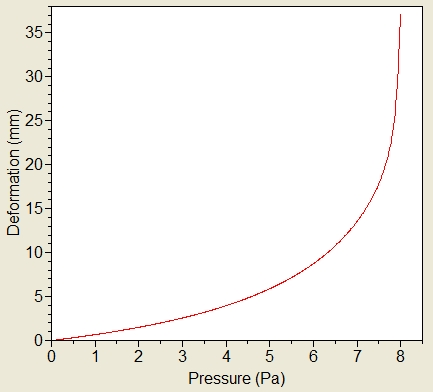In this example, even though Stabilization was turned on, I could not get the solver to converge past the 0.888 time or 7.99 Pa. [Correction made]

I tried ARCLEN for the first time and increased the pressure to 15 Pa. I see what you mean about the results. I can't do the same thing with Time as worked above.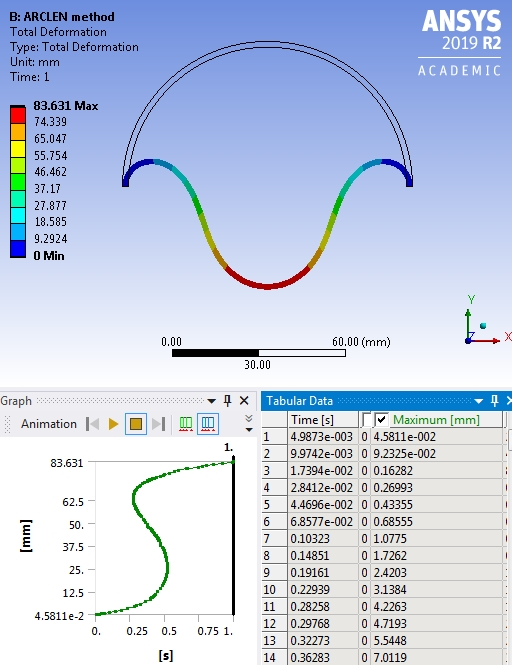•Venugopalb
Subscriber

I have some other questions.

1. How do you calculate the stabilization force for a particular structure?

2. As per you told earlier, if the problem is not converged beyond some load, it follows newton raphson method. To overcome this problem we go for ARCLEN method. Because many articles says ARCLEN method has ability to calculate post collapse behavior (But newton raphson method not, it calculates upto yield point only). What is your opinion about this?

3. The graph displacement vs Pressure plotted by you is not look like, it converged load value as 8.88 pa. I think it is approximately 8 pa. Then how do you conclude that it is 8.88 pa?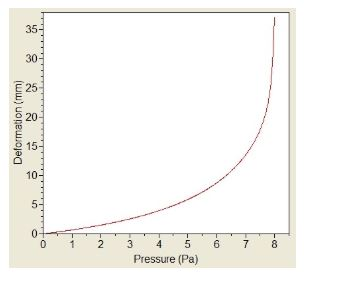•jj77
Subscriber

Nice Peter - classic example of snapthrough benchmark and in the verification manual of many software (VM17 in ansys).

Arclength: If you take the time column and multiply it by 15 (Pressure load) you get the variation of the pressure load - should be since the time is the cuts done by arc-length. Just a rough estimate it seems to snap through at 0.5 so that is about 8 Pa, which seems ball park OK looking at the stabilisation run

•Venugopalb
Subscriber

Hello jj77`!

Do you know how to plot Pressure vs Deformation (to analyse post buckling behavior) plot for an ARCLEN method?

Thanking you

•jj77
Subscriber

Read carefully what I have posted above your post and in my previous post before that

•Venugopalb
Subscriber

Sorry for inconvenience jj77

Your previous post does not give any information about how to plot this load vs deformation curve. But instead you told that the use time increment is not a correct way to plot it. Right? may be i am not properly understand your explanation.

I agree with you. time incrementation column will not worry about the ARCLEN cut backs. It only shows the time increment, whenever we us this, we does not get any snap through curve (it may form negative slope).

Can you explain me?

•peteroznewman
Subscriber

1. I used the default values for stabilization.

2. It's more correct to say that Newton-Raphson can only calculate load increments that include a static equilibrium.  So in a snap-through problem, there is no static equilibrium beyond 8 Pa, because the next increment requires less pressure, not more, but the next increment can only increase the pressure.  Now if I switch from a Pressure load to a displacement load, I can continue to increment the displacement downward, and the reaction force will reach a peak and then decrease. That is no problem and the approach I have always taken.  ARCLEN allows the solver to reduce the pressure to advance the solution through the unstable snap-through part and get onto the next part of the solution which is in static equilibrium again.

3. I made a correction in the post above.  The time was 0.888 so the pressure was 9*0.888 = 7.99 Pa.

•Venugopalb
Subscriber

Thank you peteroznewman

•jj77
Subscriber

This is what is called force vs displacement control - force control can not capture a negative portion of the curve (so beyond the flat portion), but displacements control (so when enforced displ. are used) can. Unfortunately disp. control can often not capture severe snap through type of buckling, but arclength method can.

•Venugopalb
Subscriber

Yes. ARCLEN can capture severve snap through type of buckling. In force control methods we use time increment as a multiplication factor to calculate buckling load. Likewise, If we used an ARCLEN method, any factors are there to calculate buckling load and plot the load vs displacement curve?

Thanking you

•jj77
Subscriber

I made this post to explain to you how to do this (calculate applied load with arclen) - if you have not seen it is here

https://forum.ansys.com/forums/topic/arclen-force-displacement-curve/

•peteroznewman
Subscriber

Ah, I didn't notice it above on my first time using ARCLEN, but time goes backward during the solution!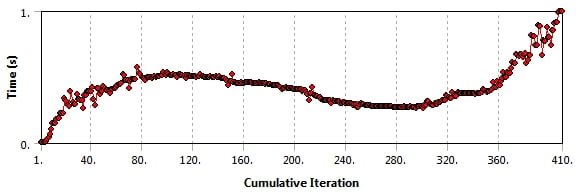So it turns out I can use the same method of multiplying time by the end pressure as I do for non ARCLEN, which is the same as what jj77 said in his post.

•Venugopalb
Subscriber

yes.

This is correct jj77 and peteroznewman.

Thank you for your knowledge sharing.

I will try it today and will be there for further discussion.

•Venugopalb
Subscriber

Hello Peter and jj77!

I have done the linear buckling analysis of an ellipsoidal dome in Ansys workbench and it gives the buckling load of 17.5 MPa. Then I added geometric imperfection of 0.1% and material non-linearity to the model. I ran the non-linear buckling analysis, the time factor linearly increasing, there are no cutbacks occur in the ARCLEN method. It means, it gives the same buckling load, what I got in the linear buckling analysis. I have attached some of the details of the model.

•Venugopalb
Subscriber

jj77 sorry for the late reply.

I have switched the large deformation key in my model. Earlier I tried bilinear material model, also used multilinear plasticity data in my model.

Is any problem with this model. I have attached the multilinear material data with this.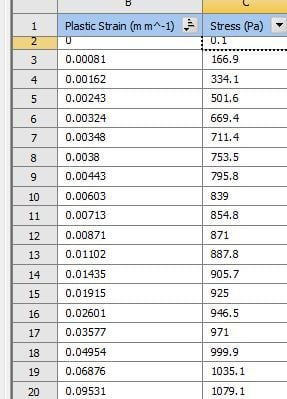I don't know how to import my model here. Can u suggest to upload it?

Thanking you

•jj77
Subscriber

In Ansys WB, go to File/Archive first. That will save a wbpz file which you can now attach. To do that:

Then look on your post above and to the right there is a button called Attach (amongst others like Edit). Press that and attach.

•Venugopalb
Subscriber

jj77

I tried it. Here only image and web page link can be uploaded, it does not accept the files.

There is no such option to upload the model here. If u give your mail I will send it to you. Is any other option available?

•jj77
Subscriber

So save a wbpz file by File/Archive and use the attach button in your above post - people have done that many times before here.

I just did it here. Look in the attached image and see how it is done.

•Venugopalb
Subscriber

jj77 Thank you

I got your point. The server is busy now. I will upload it as soon as possible.

•Venugopalb
Subscriber

Hi jj77

I have tried to upload the file, but after uploading google displays an error message "the file size is too large you cannot open this page". I think it is better, if you give your mail id, I will share it through a drive.

Thanking you

•Venugopalb
Subscriber

Hi jj77

I have attached the model in my previous post. Please look at this.

I don't know why the nonlinear buckling load is not lesser than the linear buckling load. Also, the deformation of the structure is very less (it is not up to the expected level).

If u find the cause it is useful for me.

Thanking you

•jj77
Subscriber

I do not use this version so I can not look at it (I use 19.1).

A suggestion because this discussion is really closed and is getting too long.

Open a new one and attach it there with your question and perhaps someone else will look at it.

•Venugopalb
Subscriber

Thank You jj77

•Venugopalb
Subscriber

Hi jj77!

One last question, We are adding imperfection scale factor as 0.1,0.5,1.0 like this. This is in percentage or we have multiplied by it 100?

Is it possible to visualize those imperfections that we added in Ansys?

•jj77
Subscriber

so it is factor * deformations.